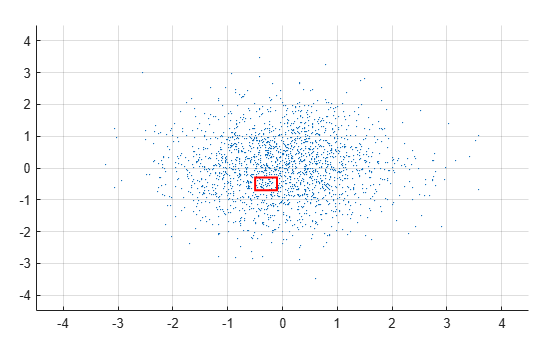## Customized Presentations and Special Effects with Tiled Chart Layouts

A tiled chart layout lets you control the placement of elements in a visualization and create special effects. This topic covers four examples:

### Stacked Colorbar and Plot with Shared Title

Tiled chart layouts have several features for presenting multiple elements together under a shared title. In this case, stack a colorbar on top of a plot under a shared title.

Create a `1`-by-`1` tiled chart layout. Then display a contour plot of the `peaks` data set.

```figure t = tiledlayout(1,1); nexttile contourf(peaks)```Create a colorbar, and specify the `'northoutside'` location. Then add a shared title by passing the layout object, `t`, to the `title` function.

```cb = colorbar('Location','northoutside'); title(t,'Contours of Peaks')```### Irregular Grid of Plots

Tiled chart layouts can be nested. This is useful for varying the arrangement of the tiles in a layout. In this case, create two columns that each have a different number of tiles.

Create a 1-by-2 tiled layout called `t1`. Then create two nested layouts, `t2` and `t3`, where `t2` is in the first tile of `t1`, and `t3` is in the second tile. Then display two contour plots in `t2`, and add a title above the plots.

```figure t1 = tiledlayout(1,2,'TileSpacing','Compact'); t2 = tiledlayout(t1,'flow','TileSpacing','Compact'); t3 = tiledlayout(t1,'flow','TileSpacing','Compact'); t3.Layout.Tile = 2; % Add two contour plots to left side [X,Y,Z] = peaks; nexttile(t2); contourf(X,Y,Z) nexttile(t2) contourf(X,Y,Z,10) title(t2,"Contours of Peaks")```Display three line plots within `t3`, and add a title.

```nexttile(t3) plot(Z(15,:)) nexttile(t3) plot(Z(25,:)) nexttile(t3) plot(Z(35,:)) title(t3,"Y = -1.25, 0, and 1.25")```### Main Plot with Adjacent Smaller Plots

A tiled chart layout consists of a grid of tiles surrounded by four outer tiles. You can place legends, colorbars, additional axes, or even a nested layout into any of these tiles. In this case, create a main plot in the center, and use one of the outer tiles to display a set of supplemental plots.

First, create a matrix of sine waves and plot them together in a `1`-by-`1` tiled chart layout.

```x = (0:0.1:10)'; y = sin([x x+1 x+2 x+3 x+4 x+5]); figure t = tiledlayout(1,1); nexttile plot(x,y)```Plot the individual sine waves below the main plot. To do this, create a new layout called `ts` in the south tile of layout `t`. When you create `ts`, specify the `'flow'` tile arrangement so that the plots fill the entire south tile at each iteration of the `for` loop.

```ts = tiledlayout(t,'flow'); ts.Layout.Tile = 'south'; for i=1:5 nexttile(ts); plot(x,y(:,i)) end```### Region-of-Interest Plot

A plot can span multiple tiles of a layout. You can use this feature to display a main plot with an accompanying inset plot. In this case, the inset plot shows a zoomed-in view of a region of interest.

Create a 3-by-3 tiled chart layout, and create an axes that spans all of the tiles. Then display a scatter plot in the axes.

```figure t = tiledlayout(3,3,'Padding','compact'); ax1 = nexttile(1,[3 3]); x = randn(2000,1); y = randn(2000,1); scatter(ax1,x,y,1,'filled');```Next, create a rectangle that defines the region of interest in the scatter plot. Set properties on the axes last, to ensure that those properties persist.

• Define the bounds of the rectangle. Define `left` and `bottom` as the left and bottom edges of the rectangle. Specify `left` in x-axis units, and specify `bottom` in y-axis units. Similarly, define `width` and `height` as the width and height of the rectangle, also in axis units.

• Display the rectangle. Call the `hold` function to preserve the contents of the axes, and then call the `rectangle` function.

• Set properties on the axes. Set the font size to `10` points, set the x- and y-axis limits, and turn the grid on.

```% Define bounds of the rectangle left = -0.5; bottom = -0.7; width = 0.4; height = 0.4; % Display the rectangle hold(ax1,'on'); r = rectangle('Position',[left bottom width height], ... 'EdgeColor','red','LineWidth',1.5); % Set properties on the axes ax1.FontSize = 10; ax1.XLim = [-4.5 4.5]; ax1.YLim = [-4.5 4.5]; grid(ax1,'on')```Display a smaller, zoomed-in plot with x- and y-axis limits that match the region of interest.

• Create the axes for the zoomed-in view. Call the `axes` function to create the axes. Move the axes to the third tile by setting the `Layout.Tile` property. Then plot the entire set of x and y data.

• Adjust the axis limits to match the region of interest. Set the `XLim` and `YLim` properties of `ax2` to match the region of interest. Then remove the ticks from the plot box.

• Set other properties on the axes. Turn the axes box on, set the x- and y- axis colors to red, and display a title.

```% Create axes for zoomed-in view ax2 = axes(t); ax2.Layout.Tile = 3; scatter(ax2,x,y,10,'filled'); % Adjust axis limits and remove ticks ax2.XLim = [left left+width]; ax2.YLim = [bottom bottom+height]; ax2.XTick = []; ax2.YTick = []; % Set other properties on the axes ax2.Box = 'on'; ax2.XAxis.Color = 'red'; ax2.YAxis.Color = 'red'; title(ax2,'100x Magnification','Color','red');```